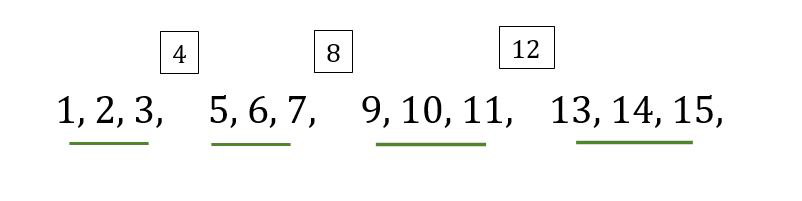# Find the Nth natural number which is not divisible by A

Given two integers A and N, our task is to find the Nth natural number which is not divisible by A.

Examples:

Input: A = 4, N = 12
Output: 15
Explanation:
The series starting from 1 excluding the multiples of A would be 1, 2, 3, 5, 6, 7, 9, 10, 11, 13, 14, 15, 17 and the 12th term which is not divisible by 4 is 15.

Input: A = 3, N = 20
Output: 29
Explanation:
The series starting from 1 excluding the multiples of A would be 1, 2, 4, 5, 7, 8, 10, 11 and so on and the Nth number which is not divisible by 3 is 29.

## Recommended: Please try your approach on {IDE} first, before moving on to the solution.

Approach:

To solve the problem mentioned above we have to observe that there is a gap after every (A – 1) integer as the multiples of A are skipped. To find that the number N lies in which set we divide N by (A – 1), and store it in a variable lets say quotient. Now multiplying A with that variable and adding the remainder to it we will get the resultant answer.

```If A = 4, N = 7:
quotient = 7 / 3 = 2
remainder = 7 % 3 = 1

(A * quotient) + remainder
= 4 * 2 + 1 = 9
```But if the remainder is 0 it means it is the last element in the set. Lets us understand it by an example.

```If A = 4, N = 6:
quotient = 6 / 3 = 2
remainder = 6 % 3 = 0

(A * quotient) - 1
= 4 * 2 - 1 = 7
```

Below is the implementation of the above approach:

## C++

 `// C++ code to Find the Nth number which ` `// is not divisible by A from the series ` `#include ` `using` `namespace` `std; ` ` `  `void` `findNum(``int` `n, ``int` `k) ` `{ ` `     `  `    ``// Find the quotient and the remainder ` `    ``// when k is divided by n-1 ` `    ``int` `q = k / (n - 1); ` `    ``int` `r = k % (n - 1); ` `    ``int` `a; ` ` `  `    ``// If the remainder is not 0 ` `    ``// multiply n by q and subtract 1 ` `    ``// if remainder is 0 then ` `    ``// multiply n by q ` `    ``// and add the remainder ` `    ``if``(r != 0) ` `       ``a = (n * q) + r; ` `    ``else` `       ``a = (n * q) - 1; ` ` `  `    ``// Print the answer ` `    ``cout << a; ` `} ` ` `  `// Driver code ` `int` `main() ` `{ ` `    ``int` `A = 4, N = 6; ` `     `  `    ``findNum(A, N); ` `    ``return` `0; ` `} ` ` `  `// This code is contributed by PratikBasu `

## Java

 `// Java code to Find the Nth number which ` `// is not divisible by A from the series ` `class` `GFG { ` `     `  `static` `void` `findNum(``int` `n, ``int` `k) ` `{ ` ` `  `    ``// Find the quotient and the remainder ` `    ``// when k is divided by n-1 ` `    ``int` `q = k / (n - ``1``); ` `    ``int` `r = k % (n - ``1``); ` `    ``int` `a = ``0``; ` `     `  `    ``// If the remainder is not 0 ` `    ``// multiply n by q and subtract 1 ` `    ``// if remainder is 0 then ` `    ``// multiply n by q ` `    ``// and add the remainder ` `    ``if` `(r != ``0``) ` `        ``a = (n * q) + r; ` `    ``else` `        ``a = (n * q) - ``1``; ` ` `  `    ``// Print the answer ` `    ``System.out.println(a); ` `} ` ` `  `// Driver code ` `public` `static` `void` `main(String[] args) ` `{ ` `    ``int` `A = ``4``; ` `    ``int` `N = ``6``; ` ` `  `    ``findNum(A, N); ` `} ` `} ` ` `  `// This code is contributed by 29AjayKumar `

## Python3

 `# Python code to Find the Nth number which ` `# is not divisible by A from the series ` ` `  `def` `findNum(n, k): ` `     `  `    ``# Find the quotient and the remainder ` `    ``# when k is divided by n-1 ` `    ``q ``=` `k``/``/``(n``-``1``) ` `    ``r ``=` `k ``%` `(n``-``1``) ` `     `  `    ``# if the remainder is not 0  ` `    ``# multiply n by q and subtract 1 ` `     `  `    ``# if remainder is 0 then  ` `    ``# multiply n by q  ` `    ``# and add the remainder ` `    ``if``(r !``=` `0``): ` `        ``a ``=` `(n ``*` `q)``+``r ` `    ``else``: ` `        ``a ``=` `(n ``*` `q)``-``1` `         `  `    ``# print the answer ` `    ``print``(a) ` ` `  `# driver code ` `A ``=` `4` `N ``=` `6` `findNum(A, N) `

Output:

```7
```My Personal Notes arrow_drop_upCheck out this Author's contributed articles.

If you like GeeksforGeeks and would like to contribute, you can also write an article using contribute.geeksforgeeks.org or mail your article to contribute@geeksforgeeks.org. See your article appearing on the GeeksforGeeks main page and help other Geeks.

Please Improve this article if you find anything incorrect by clicking on the "Improve Article" button below.

Improved By : 29AjayKumar, PratikBasu